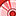# QlikView App Dev

Discussion Board for collaboration related to QlikView App Development.

Announcements
QlikWorld 2023, a live, in-person thrill ride. Save \$300 before February 6: REGISTER NOW!
cancel
Showing results for
Did you mean:Contributor

## How to match the product name with an expression value

Hello all,

I'm hoping someone can help me out with something I've been struggling on. I am trying to get the name in a text box of the product with the worst variance year to date against budget. I can get the variance using the formula below. This basically looks at each month in the year and if the current month is greater or equal to it, it sums it and then compares it against actuals. The aggr groups it by brand and then the min takes the smallest one. The formula returns -3.18 which is the largest variance of the brands but I need it to return the brand's name. I'm thinking the match() function could be of use here but I can't get it to work... any thoughts?

=min(

aggr((sum({<Scenario={'\$(vCscenario)'},M={'S'}>}Salesm)/1000000)-

((sum({<M={'S'},s.Month={1}>}Salesm)/1000000

+ if(\$(vMaxMonth)>1,sum({<M={'S'},s.Month={2}>}Salesm)/1000000,0)

+ if(\$(vMaxMonth)>2,sum({<M={'S'},s.Month={3}>}Salesm)/1000000,0)

+ if(\$(vMaxMonth)>3,sum({<M={'S'},s.Month={4}>}Salesm)/1000000,0)

+ if(\$(vMaxMonth)>4,sum({<M={'S'},s.Month={5}>}Salesm)/1000000,0)

+ if(\$(vMaxMonth)>5,sum({<M={'S'},s.Month={6}>}Salesm)/1000000,0)

+ if(\$(vMaxMonth)>6,sum({<M={'S'},s.Month={7}>}Salesm)/1000000,0)

+ if(\$(vMaxMonth)>7,sum({<M={'S'},s.Month={8}>}Salesm)/1000000,0)

+ if(\$(vMaxMonth)>8,sum({<M={'S'},s.Month={9}>}Salesm)/1000000,0)

+ if(\$(vMaxMonth)>9,sum({<M={'S'},s.Month={10}>}Salesm)/1000000,0)

+ if(\$(vMaxMonth)>10,sum({<M={'S'},s.Month={11}>}Salesm)/1000000,0)

+ if(\$(vMaxMonth)>11,sum({<M={'S'},s.Month={12}>}Salesm)/1000000,0))),Brand))

0 RepliesCommunity Browser